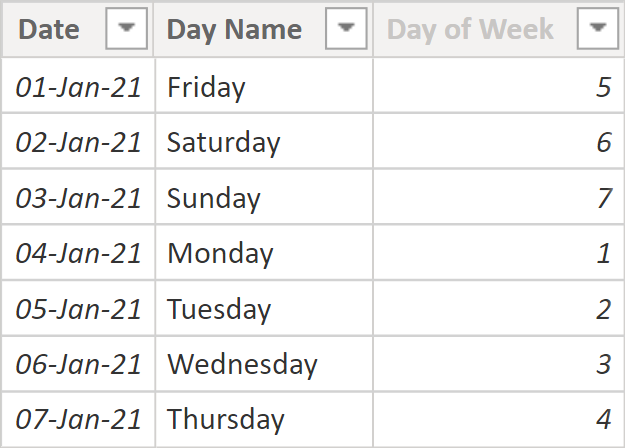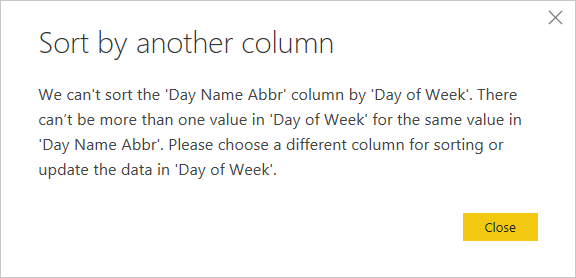# Single-letter day and month names in Power BIOccasionally in Power BI, you may want to display day or month names as single letters to save space. This may result in duplicates because neither day nor month names are unique when you shorten them to one letter. In this blog post, I’m showing two solutions to the problem: one in DAX and one in Power Query (M language).

### The problem

Let’s say you’ve got a calendar table as follows:The table can be created in DAX by using the following formula:

``````Calendar =
CALENDAR("2021-01-01", "2021-01-07"),
"Day Name", FORMAT([Date], "dddd"),
"Day of Week", WEEKDAY([Date], 2)
) ``````

Here’s the corresponding M code:

``````#table(
type table [Date=date, Day Name=text, Day of Week=Int64.Type],
List.Transform(
List.Dates(#date(2021, 1, 1), 7, #duration(1, 0, 0 ,0)),
each {_, Date.DayOfWeekName(_), Date.DayOfWeek(_)}
)
)``````

Now let’s say you want to shorten day names to single letters: Friday should be F, Saturday should be S, and so on.

In DAX, you could add the following calculated column:

``Day Name Abbr = LEFT('Calendar'[Day Name], 1)``

In M, you could use the following custom column:

``Text.Start([Day Name], 1)``

Either way, if you attempt to sort the new column by the Day of Week column, you’ll get the following error:This is because for each single-letter day name, there may be more than one Day of Week value, like for letter S: 6 and 7. With month names, you’ll run into the same problem. Therefore, we’ve got to make the names unique.

### The solution in DAX

The trick is to add some non-printing characters to distinguish the values. For example, you can use zero-width space: `UNICHAR(8203)`. To make the values different, add a different number of zero-width spaces to each letter like so:

``````Day Name Abbr =
CONCATENATE(
LEFT('Calendar'[Day Name], 1),
REPT(UNICHAR(8203), 'Calendar'[Day of Week])
)``````

`REPT` repeats a text string — exactly what we need.

### The solution in Power Query (M)

The corresponding custom column formula in M language is

``Text.Start([Day Name], 1) & Text.Repeat(Character.FromNumber(8203), [Day of Week])``

Now that for each single-letter day name there’s only one Day of Week value, we can sort the new column by Day of Week.

Note: strictly speaking, one-to-one relationship between a column and a sorting column is not necessary; refer to Sort by Column in Power BI for more details.

Sample file: Single-letter day month.pbix (40 KB)